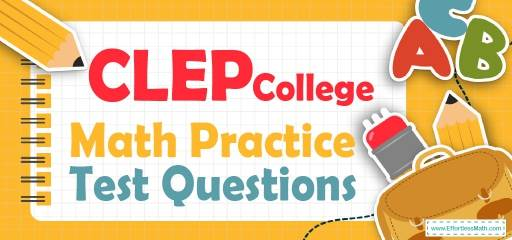# CLEP College Math Practice Test QuestionsPreparing students for the CLEP College Math test? Try these free CLEP College Math Practice questions. Reviewing practice questions is the best way to brush up on your student’s Math skills. Here, we walk you through solving 10 common CLEP College Math practice problems covering the most important math concepts on the CLEP College Math test.

These CLEP College Math practice questions are designed to be similar to those found on the real CLEP College Math test. They will assess your students’ level of preparation and will give them a better idea of what to study on their exams.

## 10 Sample CLEP College Math Practice Questions

1- A bag contains 18 balls: two green, five black, eight blue, a brown, a red, and one white. If 17 balls are removed from the bag at random, what is the probability that a brown ball has been removed?

A. $$\frac{1}{9}$$

B. $$\frac{1}{6}$$

C. $$\frac{16}{18}$$

D. $$\frac{17}{18}$$

2- The average of five consecutive numbers is 36. What is the smallest number?

A. 38

B. 36

C. 34

D. 12

3- The price of a car was $28,000 in 2012. In 2013, the price of that car was$18,200. What was the rate of depreciation of the price of a car per year?

A. $$20\%$$

B. $$30\%$$

C. $$35\%$$

D. $$40\%$$

4- The width of a box is one-third of its length. The height of the box is one-third of its width. If the length of the box is 36 cm, what is the volume of the box?

A. $$81 cm^3$$

B. $$162cm^3$$

C. $$243cm^3$$

D. $$1,728cm^3$$

5- A tree 32 feet tall casts a shadow 12 feet long. Jack is 6 feet tall. How long is Jack’s shadow?

A. 2.25 feet

B. 4 feet

C. 4.25 feet

D. 8 feet

6- When a number is subtracted from 28 and the difference is divided by that number, the result is 3. What is the value of the number?

A. 2

B. 4

C. 7

D. 12

7- An angle is equal to the one-ninth of its supplement. What is the measure of that angle?

A. 9

B. 18

C. 25

D. 60

8- John traveled 150 km in 6 hours and Alice traveled 140 km in 4 hours. What is the ratio of the average speed of John to the average speed of Alice?

A. 3∶2

B. 2∶3

C. 5∶7

D. 5∶6

9-If A={2,5,11,15}, B={1,2,3,4,5,6}, and C={5,7,9,11,13}, then which of the following set is $$(A∪B)∩C$$?

A. {1,2,3,4,5,6,11,15}

B.{1,2,3,4,5,6,7,11,13,15}

C. {5,11,13,15}

D. {5,11}

10- If $$4n-3≥1$$, what is the least possible value of $$4n+3$$?

A. 3

B. 4

C. 7

D. 9

## Best CLEP College Math Prep Resource for 2022

1- D
If 17 balls are removed from the bag at random, there will be one ball in the bag. The probability of choosing a brown ball is 1 out of 18. Therefore, the probability of not choosing a brown ball is 17 out of 18 and the probability of having not a brown ball after removing 17 balls is the same.

2- C
Let $$x$$ be the smallest number. Then, these are the numbers: $$x, x+1, x+2, x+3, x+4$$
average$$=\frac{sum \ of \ terms }{number \ of \ terms}⇒36=\frac{x+(x+1)+(x+2)+(x+3)+(x+4)}{5}⇒36=\frac{5x+10}{5 }⇒$$
$$180=5x+10 ⇒170= 5x⇒x=34$$

3- C
Use this formula: Percent of Change: $$\frac{New \ Value-Old \ Value}{Old \ Value}×100\%$$ .
$$\frac{18,200-28,000}{28,000}×100\%=-35\%$$. The negative sign means that the price decreased

4- D
If the length of the box is 36, then the width of the box is one-third of it, 12, and the height of the box is 4 (one-third of the width). The volume of the box is: $$V=lwh=(36)(12)(4)=1,728$$

5- A
Write a proportion and solve for the missing number. $$\frac{32}{12}=\frac{6}{x}→32x=6×12=72$$
$$32x=72→x=\frac{72}{32}=2.25$$

6- C
Let $$x$$ be the number. Write the equation and solve for $$x$$. $$(28-x)÷x= 3$$
Multiply both sides by $$x. (28-x)= 3x$$, then add $$x$$ both sides. $$28=4x$$, now divide both sides by $$4. x=7$$

7- B
The sum of supplement angles is 180. Let $$x$$ be that angle. Therefore, $$x+9x=180$$
$$10x=180$$, divide both sides by $$10: x=18$$

8- C
The average speed of john is: 150÷ 6=25, The average speed of Alice is: 140÷4=35
Write the ratio and simplify. 25: 35 ⇒ 5∶7

9- D
The union of A and B is: $$A∪B$$={1,2,3,4,5,6,11,15}
The intersection of $$(A∪B)$$ and C is: $$(A∪B)∩C={5,11}$$

10- C
Adding 6 to each side of the inequality $$4n – 3 ≥ 1$$ yields the inequality $$4n + 3 ≥ 7$$. Therefore, the least possible value of $$4n + 3$$ is 7.

Looking for the best resource to help you succeed on the CLEP College Math test?

## The Best Books to Ace the CLEP College Math Test

### What people say about "CLEP College Math Practice Test Questions - Effortless Math: We Help Students Learn to LOVE Mathematics"?

No one replied yet.

X
52% OFF

Limited time only!

Save Over 52%

SAVE $40 It was$76.99 now it is \$36.99